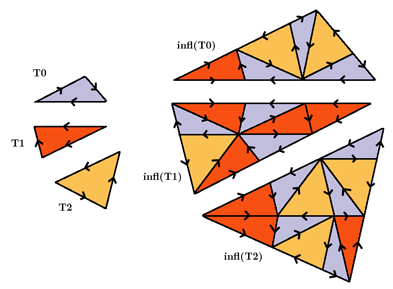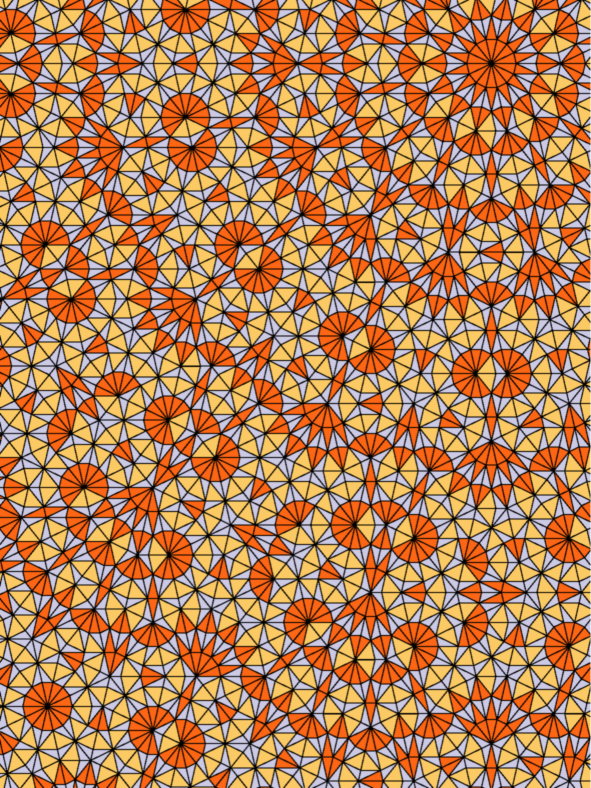## Danzer's 7-fold original

### Info

A tiling based on 7-fold (resp. 14-fold) symmetry [ND96]. The inflation factor is $1+{\sin(\frac{2\pi}{7})}/{\sin(\frac{\pi}{7})}$. The three different edge lengths are proportional to $\sin(\frac{\pi}{7})$, $\sin(\frac{2\pi}{7})$, $\sin(\frac{3\pi}{7})$. On a first glance, there seems to exist a centre of perfect 14-fold symmetry: a 14-tipped star in the upper right corner. But in fact it is only 2-fold symmetric. The symmetry is broken by the right- and left-handedness of the tiles. On rings around the 14-tipped star, this manifests in tiles pointing clockwise or counterclockwise, thus breaking the symmetry.

This is one of the rare cases that a substitution has local matching rules without decoration. In fact, the list of all different vertex stars defines the matching rule.

### Substitution Rule### Patch### References

[ND96]
Nischke, K-P and Danzer, L
A construction of inflation rules based on $n$-fold symmetry
Discrete and Computational Geometry 1996, 15,2, pp. 221-236, 96j:52035